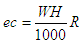# Electricity Cost Calculator

 We’ll now implement a kind of electricity cost calculator with Matlab... This calculator will help you estimate the cost of operating any given electrical device or appliance at home, based on the average kilowatt-hours (KWH) used, and on the rate (per kilowatt-hour) charged by the company that supplies you with electricity.

You need to enter the estimated power consumed by the device (in watts), the time of use (in hours) and the electricity rate (in dollars, or in your own currency, per KWH).

The calculation is based on this formula:where:
ec = electricity charges
W = power of device (in watts)
H = time (in hours)
R = electricity rate (in dollars per KWH)

The power of the device is usually mentioned somewhere on your device. Often, it has a label showing the watts (power) it consumes when in use.
Sometimes, the power is not mentioned, but the voltage (in volts, V) and current (in amperes, A) are mentioned. Power equals volts multiplied by amperes (W = VA).

We then create an easy Matlab function to calculate the formula above:

function ec = electric_cost_calc(w, h, r)
ec = w * h/1000 * r;

We create another script to drive the calculator (let's call this driver try_ec.m):

clc; clear; close all; format bank; format compact

w = input('Enter power of your device (in watts): ');
h = input(
'Enter time (in hours): ');
r = input(
'Enter electricity rate (in dollars per KWH): ');

ec = electric_cost_calc(w, h, r)

To find out how much your lighting costs are, enter the number of bulbs you use, then choose a bulb type (in watts). Light bulb wattage is usually printed on the bulb. Then you enter the time of use and the rate you’re being charged (per KWH, quoted by your electricity company).

Example 1:

You’ve been using 6 60W ligth bulbs for 3 hours. Your electricity rate is 13.1 cents per KWH. What’s the charge for this consumption of electricity?

We launch our handy electricity-cost-calculator and enter try_ec (or the name we saved for our driver) on our command window.

Enter power of your device (in watts): 6 * 60
Enter time (in hours): 3
Enter electricity rate (in dollars per KWH): 0.131

The result is:

ec = 0.14
(that is 14 cents)

Example 2:

You’re experimenting with scientific devices at home. Your great invention consumes 1200 W during 30 minutes. The assumed electricity charges from your company are 16.3 cents per KWH. How much does testing your invention cost you?

We launch again our calculator and get:

Enter power of your device (in watts): 1200
Enter time (in hours): .5
Enter electricity rate (in dollars per KWH): .163

The result is:

ec = 0.10 (that is 10 cents)

Now, knowing how to calculate electricity consumption is the first step to  energy savings in the future, right?

From 'Electricity Cost Calculator' to home

From 'Electricity Cost Calculator' to 'Matlab Examples'